# Consider 3 jointly discrete andom variables X, Y, Z whose joint PMF is given by p(x,...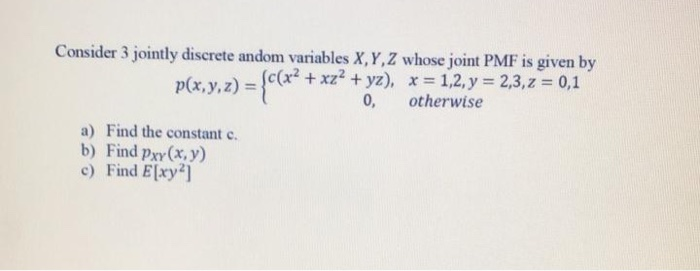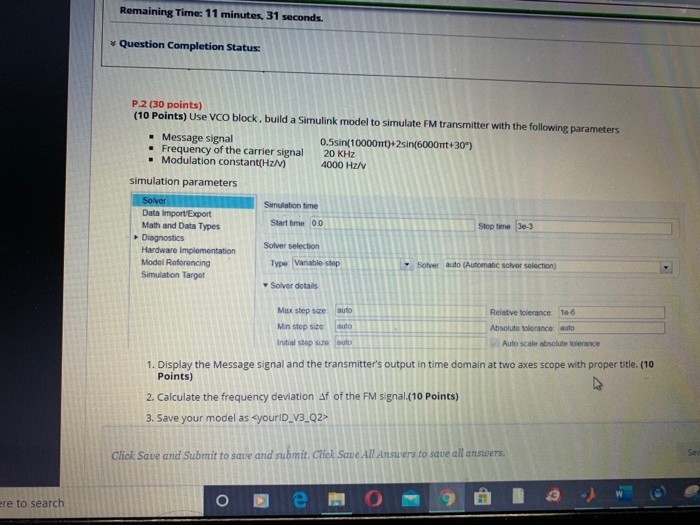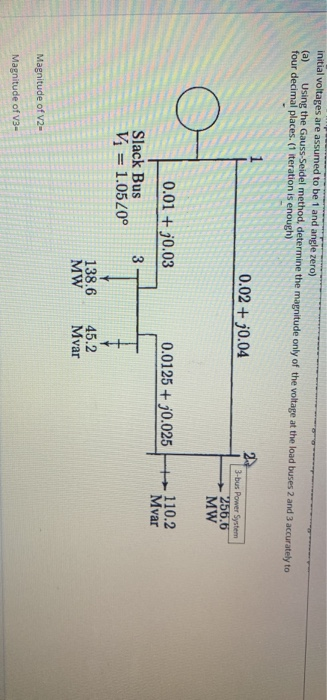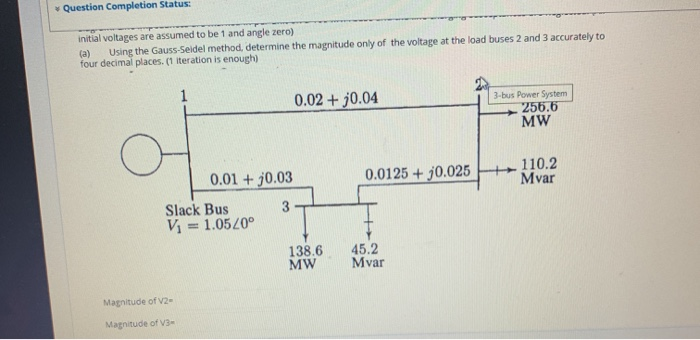Consider 3 jointly discrete andom variables X, Y, Z whose joint PMF is given by p(x, y, z) = {((x+ + xz? + yz), x = 1,2, y = 2,3, z = 0,1 0, otherwise a) Find the constant c. b) Find Pxy(x, y) c) Find E[xy?)
Remaining Time: 11 minutes, 31 seconds. Question Completion Status P.2 (30 points) (10 Points) Use VCO block, build a Simulink model to simulate FM transmitter with the following parameters - Message signal 0.5sin(10000mt)+2sin(6000m+30°) • Frequency of the carrier signal 20 KHz . Modulation constant(Hz/V) 4000 Hz simulation parameters Solver Simulation time Data ImportExport Math and Data Types Start time 001 Stop time 30-3 Diagnostics Solver selection Hardware Implementation Model Referencing Type Variable stop Soyer auto (Automatic solver selection) Simulation Target Solver details Max step size auto Min stop size auto Initial stepsze Buto Relative tolerance: 10-6 Absolute tolerance auto Auto scale absolute tolerance 1. Display the Message signal and the transmitter's output in time domain at two axes scope with proper title. (10 Points) 2. Calculate the frequency deviation Af of the FM signal.(10 Points) 3. Save your model as yourID_V3_Q2> Click Save and Submit to save and submit. Click Save All Answers to save all answers. ere to search o De 0912W
initial voltages are assumed to be 1 and angle zero) (a) Using the Gauss-Seidel method, determine the magnitude only of the voltage at the load buses 2 and 3 accurately to four decimal places. (1 iteration is enough) 0.02 + 30.04 3-bus Power System - 256.6 MW 0.01 + 0.03 0.0125+ 20.025 110.2 Mvar Slack Bus Vi = 1.05 20° 138.6 MW 45.2 Mvar Magnitude of V2- Magnitude of V3-
initial voltages are assumed to be 1 and angle zero) (a) Using the Gauss-Seidel method, determine the magnitude only of the voltage at the load buses 2 and 3 accurately to four decimal places. (1 iteration is enough) 0.02 + 30.04 3-bus Power System 256,6 MW 0.01 + 30.03 0.0125 + 0.025 + 110.2 Mvar 3- Slack Bus Vi = 1.0520° 138.6 MW 45.2 Mvar Magnitude of V2- Magnitude of V3-

We would be looking at the first question, all parts here as:

a) The probabilities for each combination of values of X, Y and Z here are computed as:

 x y z p(x, y, z) 1 2 0 c 1 2 1 4c 1 3 0 c 1 3 1 5c 2 2 0 4c 2 2 1 8c 2 3 0 4c 2 3 1 9c

Sum of all probabilities should be 1. Therefore, we have here:
c + 4c + c + 5c + 4c + 8c + 4c + 9c = 1

36c = 1

Therefore 1/36 is the required value of c here.

b) The PDF here is obtained from the above table as:

p(x = 1, y = 2) = p(x = 1, y = 2, z = 0) + p(x = 1, y = 2, z = 1) = 5c = 5/36

Similarly all other probabilities are obtained and PDF here is given as:

p(1,2) = 5/36
p(1,3) = 6/36 = 1/6
p(2,2) = 12/36 = 1/3
p(2,3) = 13/36

This is the required joint PDF for XY

c) The expected value of xy2 here is computed as: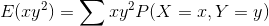E(xy2) = 4*(5/36) + 9*(1/6) + 8*(1/3) + 18*(13/36) = (5/9) + (3/2) + (8/3) + (13/2) = 8 + (29/9) = (101)/9

Therefore (101)/9 is the required expected value here.

##### Add Answer to: Consider 3 jointly discrete andom variables X, Y, Z whose joint PMF is given by p(x,...
Similar Homework Help Questions
• ### Q2. i) The one-line diagram of simple three-bus power system with generation at bus 1 is shown in figure Q2. 0.02 + 30.04 2 256.6 MW 0.0125 + 30.025 +110.2 Mvar 0.01 + 30.03 Slack Bus 3 Vi = 1.0520° 138.6 MW 45.2 Mvar Figure Q2 The magnitude of voltage at

Q2. i) The one-line diagram of simple three-bus power system with generation at bus 1 is shown in figure Q2. 0.02 + 30.04 2 256.6 MW 0.0125 + 30.025 +110.2 Mvar 0.01 + 30.03 Slack Bus 3 Vi = 1.0520° 138.6 MW 45.2 Mvar Figure Q2 The magnitude of voltage at bus 1 is adjusted to 1.05 per unit. The scheduled loads at buses 2 and 3 are as marked on the diagram. Line impedances are marked in per unit...

• ### Q2. (40) Eig. 1 shows the one-line diagram of a simple three-bus power system with generation at bus 1. The magnitude of voltage at bus 1 is adjusted to 1.05 pu. The scheduled loads at buses 2 and 3...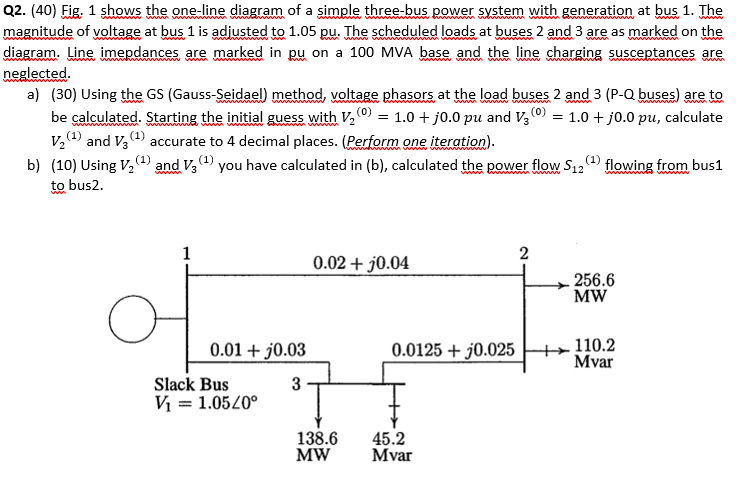Q2. (40) Eig. 1 shows the one-line diagram of a simple three-bus power system with generation at bus 1. The magnitude of voltage at bus 1 is adjusted to 1.05 pu. The scheduled loads at buses 2 and 3 are as marked on the diagram. Line imepdances are marked in pu on a 100 MVA base and the line charging susceptances are neglected a) (30) Using the GS (Gauss-Seidael) method, voltage phasors at the load buses 2 and 3 (P-Q...

• ### Consider the single line diagram of a 3-bus power system shown in Figure 2. Slack bus...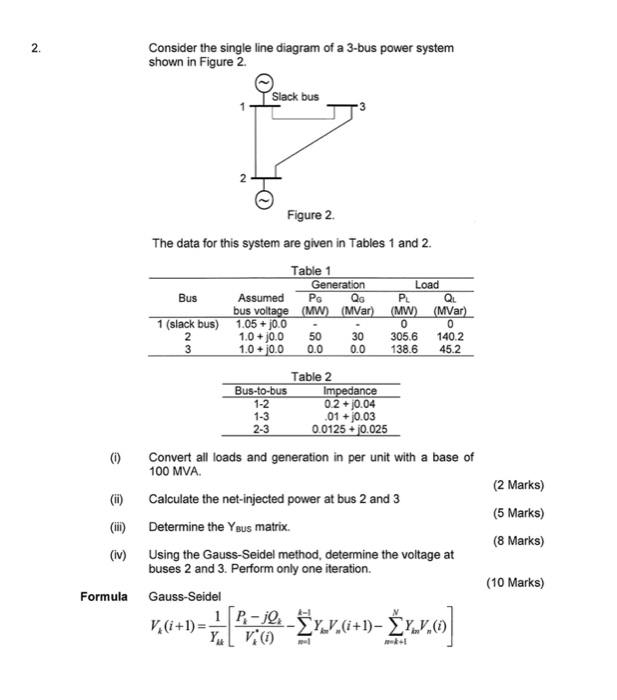Consider the single line diagram of a 3-bus power system shown in Figure 2. Slack bus 3 Figure 2. The data for this system are given in Tables 1 and 2. Bus Table 1 Generation Load Assumed PG QGPLQL bus voltage (MW) (MVar) (MW) (MVar) 1.05 +10.0 - - 1.0 + 0.0 50 30 305.6 140.2 1.0 +0.0 0.0 0.0 138.6 45.2 slack bus) Table 2 Bus-to-bus Impedance 0.2 + j0.04 .01 +0.03 2.3 0.0125 + j0.025 (0) Convert all...

• ### 3. Figure 3, shows the one-line diagram of a simple three-bus power system with generation at buses 1 and 3. The voltag...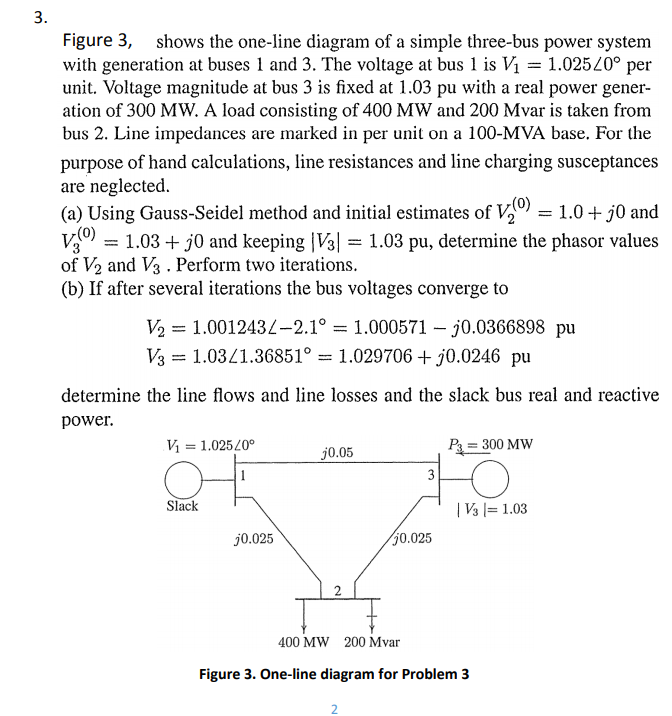3. Figure 3, shows the one-line diagram of a simple three-bus power system with generation at buses 1 and 3. The voltage at bus 1 is Vi = 1.025Z0° per unit. Voltage magnitude at bus 3 is fixed at 1.03 pu with a real power gener- ation of 300 MW. A load consisting of 400 MW and 200 Mvar is taken from bus 2. Line impedances are marked in per unit on a 100-MVA base. For the purpose of hand...

Free Homework App